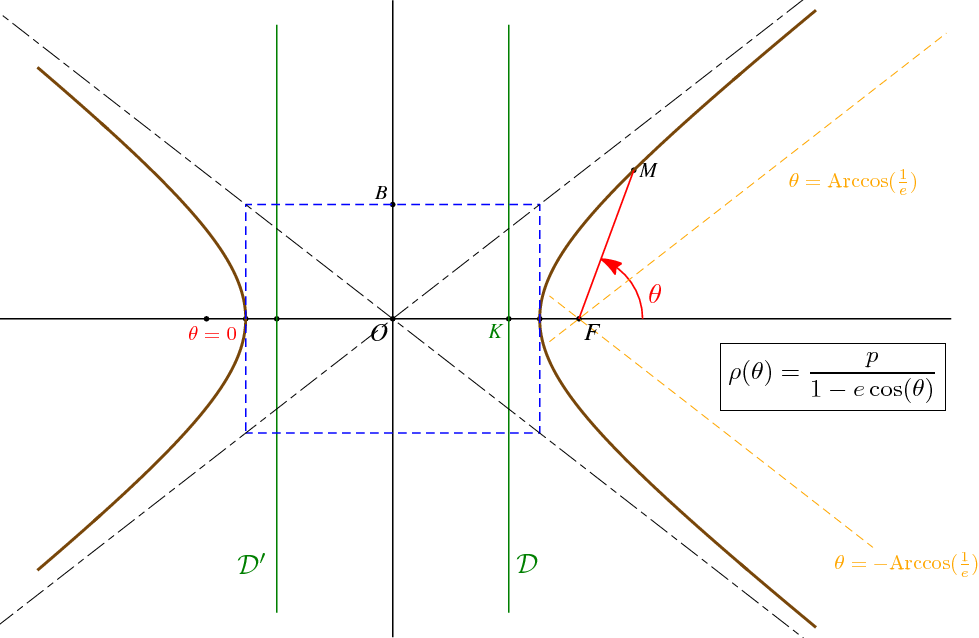Fichier 006.jps — Modifié le 26 Juin 2006 à 14 h 57Source
%% PhS
%%

35 setxunit
-8 12  setxrange
-6.5 6.5 setyrange

%%%%%%%%%%%%%%%%%%%%%%%%%%%%%%%%%%%%%%%%%%%%%%%
%% procédures
%%%%%%%%%%%%%%%%%%%%%%%%%%%%%%%%%%%%%%%%%%%%%%%

/bddroite {
8 dict begin
/kd exch def
/kg exch def
/By exch def
/Bx exch def
/B {Bx By} def
/Ay exch def
/Ax exch def
/A {Ax Ay} def
gsave
[A A B vecteur kg mulv addv A A B vecteur kd mulv addv] ligne
grestore
end
} def

/Fleche_sur_C {
2 dict begin  %% t long
/long exch def
/t exch def
[t xdet t ydet dupp dupp
t 0.01 add dup xdet exch ydet vecteur
%% 0.01 1 exch div mulv
0.01 -1 exch div mulv %% ici, il faut tricher...j'ai honte!
dupp norme long exch div mulv addv]
(->) ligne
end
} def

/Fleche_sur_Cp {
2 dict begin  %% t long
/long exch def
/t exch def
[t xdet neg t ydet dupp dupp
t 0.01 add dup xdet neg exch ydet vecteur
%% 0.01 1 exch div mulv
0.01 -1 exch div mulv %% ici, il faut tricher...j'ai honte!
dupp norme long exch div mulv addv]
(->) ligne
end
} def

%%%%%%%%%%%%%%%%%%%%%%%%%%%%%%%%%%%%%%%%%%%%%%%
%% definition des points
%%%%%%%%%%%%%%%%%%%%%%%%%%%%%%%%%%%%%%%%%%%%%%%

/a {3} def
/c {3.8} def
/b {c c mul a a mul sub sqrt} def
/e {c a div} def
/Kx {a e div} def
/K {Kx neg 0} def
/K+ {K 0 6 addv} def
/K- {K 0 -6 addv} def
/Kp {Kx 0} def
/Kp+ {Kp 0 6 addv} def
/Kp- {Kp 0 -6 addv} def
/O {0 0} def
/F {c neg 0} def
/Fp {c 0} def
/S1 {a neg 0} def
/S2 {a 0} def
/B {0 b} def

/R11 {a neg b} def
/R12 {a b} def
/R21 {a neg b neg} def
/R22 {a b neg} def
/omeg {F O milieu} def
/Ry {1 e e mul 1 exch div sub sqrt a mul} def
/R {Kx neg Ry} def

/xdet {settvar t t mul b b mul div 1 add sqrt a mul} def
/ydet {settvar t} def

/Mx {b 1.3 mul xdet} def
/My {b 1.3 mul ydet} def
/M {Mx My} def
/H {M Kp Kp+ orthoproj} def

%%%%%%%%%%%%%%%%%%%%%%%%%%%%%%%%%%%%%%%%%%%%%%%
%% definition d'une couleur
%%%%%%%%%%%%%%%%%%%%%%%%%%%%%%%%%%%%%%%%%%%%%%%

/coul1 {120 255 div 70 255 div 9 255 div setrgbcolor} def

%%%%%%%%%%%%%%%%%%%%%%%%%%%%%%%%%%%%%%%%%%%%%%%
%% tracés
%%%%%%%%%%%%%%%%%%%%%%%%%%%%%%%%%%%%%%%%%%%%%%%
1.1 setlinewidth
[O F Fp S1 S2 B K Kp M] points
F Fp -0.6 2 bddroite

coul1
2 setlinewidth
b -2.7 mul b 2.7 mul settrange
{xdet} {ydet} courbeparam
b -2.2 mul b 2.2 mul settrange
{xdet neg} {ydet} courbeparam

/arrowscale {2.5 dup} def

5 0.2 Fleche_sur_C
4 0.2 Fleche_sur_Cp
-5 0.2 Fleche_sur_C
-4 0.2 Fleche_sur_Cp

1.1 setlinewidth
vert
[K+ K-] ligne
[Kp+ Kp-] ligne

rouge
[Fp M] ligne
/arrowangle -9 def
Fp 1 0 addv Fp M 1.3 (->) tripointarc
/arrowangle 0 def

bleu
pointilles
[R11 R12 R22 R21] polygone
noir
mixte
0.6 setlinewidth
R11 R22 -1 2 bddroite
R12 R21 -1 2 bddroite

orange
pointilles
Fp Fp a b addv -0.2 2.5 bddroite
Fp Fp a neg b addv -2 0.2 bddroite

%%%%%%%%%%%%%%%%%%%%%%%%%%%%%%%%%%%%%%%%%%%%%%%
%% texte
%%%%%%%%%%%%%%%%%%%%%%%%%%%%%%%%%%%%%%%%%%%%%%%

setTimesItalic
noir
(O) O [1.8 dup] dltext
(F) Fp [1.8 dup] drtext
(B) B [1.5 dup] ultext

(M) M  [1.5 dup] crtext

vert
(K) Kp [1.5 dup] dltext

<latex>
$\mathcal{D}'$
</latex>
K 0 -5 addv [2 dup] cltexlabel

<latex>
$\mathcal{D}$
</latex>
Kp 0 -5 addv[2 dup] crtexlabel

rouge
<latex>
$\theta$
</latex>
Fp 1.6 0.5 addv [2 dup] cctexlabel

<latex>
$\theta=0$
</latex>
b neg 0 -0.7 0 addv [1.5 dup] dltexlabel

orange
<latex>
$\theta=\mathrm{Arccos}(\frac{1}{e})$
</latex>
9.4 2.8 [1.5 dup] cctexlabel

<latex>
$\theta=-\mathrm{Arccos}(\frac{1}{e})$
</latex>
10.5 -5 [1.5 dup] cctexlabel

noir
<latex>
\fbox{$\rho(\theta)=\displaystyle\frac{p}{1-e\cos(\theta)}$}
</latex>
9 -1.2 [1.8 dup] cctexlabel